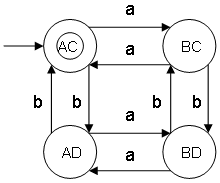# Theory of computation

The theory of computation is mathematically model a machine (for example a computer) and study the theory about it which means what are the problems which would be solved by this machine, what are the limitations of the machine etc.

## Construction of minimal DFA and cross product of DFA

YouTube: Theory Of Computation 3, Construction of DFA and cross product of DFA
YouTube: Theory Of Computation 4, DFA and problem

• Construct a minimal DFA that accepts set of all strings over {a,b} in which number of a's and b's are even
ω ∊ {a,b} and na(ω) mod 2 = 0 && nb(ω) mod 2 = 0

L = {ε,aa,bb,aabb,abab,baba,aaaa,bbbb,...}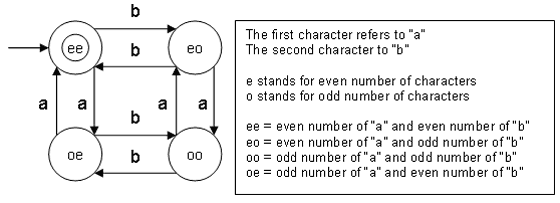Notes:
• ε is a string of length 0 and does satisfy condition na(ω) mod 2 = 0 and nb(ω) mod 2 = 0
• The language L is infinite, we do not know if the FA is finite or infinite. By drawing the FA diagram there are a finite number of states which means this minimal DFA is finite.
• na(ω) mod 2 = 0 can also be written as na(ω) 0 (mod 2).
nb(ω) mod 2 = 0 can also be written as nb(ω) 0 (mod 2).
The symbol ≅ means congruent.
• The strings are divided in four classes: "ee", "eo", "oe" and "oo".
• This minimal DFA has 4 states and state "ee" (also referred as q0) is the final state.

• Construct a minimal DFA that accepts set of all strings over {a,b} in which number of a's and b's are even using cross product
ω ∊ {a,b} and na(ω) mod 2 = 0 && nb(ω) mod 2 = 0

Counting a's

L = {ε,aa,aaaa,aaaaaa,...}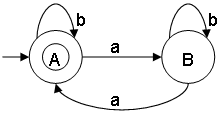Counting b's

L = {ε,bb,bbbb,bbbbbb,...}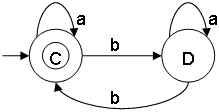Cross product (combining a's and b's)

Multiply the states {A,B} with {C,D}: {A,B} x {C,D} = {AC,AD,BC,BD}
The cross product consists of 4 states.
In counting a's, state A is the initial state.
In counting b's, state C is the initial state.
AC is the initial state of the 4 states.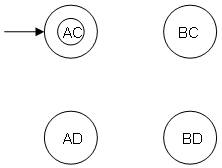Determine the transition for each state (AC,AD,BC,BD) and for each input (a,b)

• State AC and input a
Counting a's DFA diagram: A -> a -> B
Counting b's DFA diagram: C -> a -> C
Result: AC-> a -> BC
• State AC and input b
Counting a's DFA diagram: A -> b -> A
Counting b's DFA diagram: C -> b -> D
• State BC and input a
Counting a's DFA diagram: B -> a -> A
Counting b's DFA diagram: C -> a -> C
Result: BC-> a -> AC
• State BC and input b
Counting a's DFA diagram: B -> b -> B
Counting b's DFA diagram: C -> b -> D
Result: BC-> b -> BD
• State BD and input a
Counting a's DFA diagram: B -> a -> A
Counting b's DFA diagram: D -> a -> D
• State BD and input b
Counting a's DFA diagram: B -> b -> B
Counting b's DFA diagram: D -> b -> C
Result: BD-> b -> BC
• State AD and input a
Counting a's DFA diagram: A -> a -> B
Counting b's DFA diagram: D -> a -> D
• State AD and input b
Counting a's DFA diagram: A -> b -> A
Counting b's DFA diagram: D -> b -> C